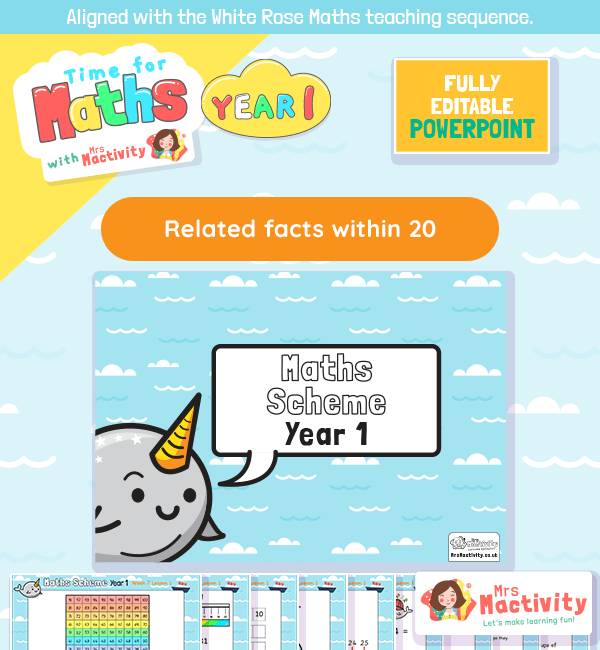Related Number Facts Lesson PresentationHelp children develop maths mastery skills with our Related Number Facts Lesson Presentation. Suitable for year 1, but adaptable for all year groups – adapt this editable related number facts PowerPoint for your class or children at home. Work on varied fluency, reasoning and problem solving skills.

National Curriculum Objectives

• Represent and use number bonds and related subtraction facts within 20
• Add and subtract one-digit and two-digit numbers to 20, including zero
• Read, write and interpret mathematical statements involving addition (+), subtraction (–) and equals (=) signs

Aligned with the White Rose Maths teaching sequence, this maths lesson presentation could be taught in Spring block 1 (addition and subtraction – related facts).

Explore our year 1 maths resources.

Explore our maths scheme.

Explore our addition and subtraction resources.